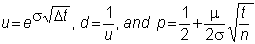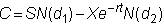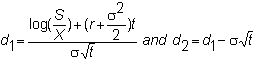﻿ 5.4 T-Note and T-Bond Futuresoffice (412) 9679367
fax (412) 967-5958
toll-free 1 (800) 214-3480

5.4  Binomial Option Pricing:  Limiting Results

 W

hat happens to the binomial option pricing model when the period of time becomes smaller and smaller?  In an important result, Cox, Ross, and Rubinstein (1979) demonstrate that the limit in the multi-period binomial option pricing model converges upon the Black-Scholes option pricing model, which was derived from a continuous time framework.

This result illustrates the power of the binomial approach for modeling option pricing problems.  By the appropriate choice of u, d, and probability p, different processes can be converged upon given different assumed structures, which makes this both a useful theoretical approach and a numerical methods tool.

In the case of the Black-Scholes model, this results when the up- and downticks and probability are modeled as:where n is the number of periods.

Under this structure, the limiting process converges upon the Black-Scholes option pricing model (see Technical Appendix to Chapter 5: Limiting Results).  This option pricing model for a European call option iswhereThe nice property that emerges from the binomial derivation of this pricing model is that the model itself can be interpreted in terms of the risk-neutral probability distributions.  That is, the first term is the expected present value of the stock conditional on the option finishing in-the-money.  The second term is the present value of the expected exercise cost.  In both cases, expectations are taken with respect to risk-neutral probabilities.

It is interesting to note the variables required to value the option in this closed form solution.  These are:  the volatility of the stock price, the risk-free rate of interest, the time to maturity, the strike price, and the stock price.  The expected return on the underlying stock is not required because only the risk-neutral probabilities are used to value the option.

Numerical Solutions

The general binomial approach can be applied to find numerical solutions to a wide range of very complicated option pricing problems.  For example, the problem of valuing an American option can be solved in a straightforward application of the binomial option pricing model by testing for the value of early exercise at each node.  The present value of the American option can then be computed as the expected value over all paths taken with respect to risk-neutral probabilities.

In Chapter 6, The Black-Scholes Option Pricing Model, we introduce the continuous time approach that Black-Scholes originally used to derive their option pricing model.